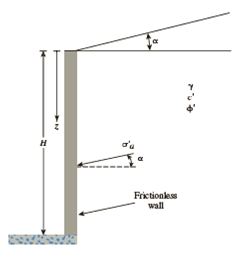Chapter 13, Problem 13.22PPrinciples of Geotechnical Enginee...

9th Edition
Braja M. Das + 1 other
ISBN: 9781305970939

Solutions

Chapter
SectionPrinciples of Geotechnical Enginee...

9th Edition
Braja M. Das + 1 other
ISBN: 9781305970939
Textbook Problem

For the data given in Problem 13.21, determine the Rankine passive force, Pp, per unit length of the wall, and its location and direction.13.21 Figure 13.13 shows a frictionless wall with a sloping granular backfill. Given: H = 7 m, α = 12°, ϕ ′ = 28°, and γ = 18.6 kN/m3.a. Determine the magnitude of active pressure, σ ′ a , at the bottom of the wall.b. Determine Rankine active force, Pa, per unit length of the wall and its location and direction.Figure 13.13 Frictionless vertical retaining wall with sloping backfill

(a)

To determine

Find the magnitude of active pressure σa at the bottom of the wall.

Explanation

Given information:

The height (H) of the retaining wall is 7.0 m.

The soil friction angle ϕ is 28°.

The angle α is 12°.

The unit weight γ of the backfill is 18.6kN/m3.

Calculation:

Determine the passive earth pressure coefficient Kp using the formula.

Kp=cosαcosα+cos2(α)cos2(ϕ)cosαcos2(α)cos2(ϕ)

Substitute 12° for α and 28° for ϕ

(b)

To determine

Find the Rankine active force Pa per unit length of the wall and its location and direction.

Still sussing out bartleby?

Check out a sample textbook solution.

See a sample solution

The Solution to Your Study Problems

Bartleby provides explanations to thousands of textbook problems written by our experts, many with advanced degrees!

Get Started

Provide suggestions for reducing input volume.

Systems Analysis and Design (Shelly Cashman Series) (MindTap Course List)

What does a program specification package identify? (524)

Enhanced Discovering Computers 2017 (Shelly Cashman Series) (MindTap Course List)

Which Cisco command lists configured VLANs on a switch?

Network+ Guide to Networks (MindTap Course List)

Can all electrodes be used with a leading angle? Why or why not?

Welding: Principles and Applications (MindTap Course List)